### Spun (DDA)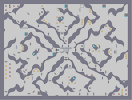Hover over the thumbnail for a full-size version.

Author kendogg102 author:kendogg102 dda rated 2006-03-09 2006-03-09 4 by 7 people. \$Spun (DDA)#kendogg102#none#00;=0000007900000000007006<00000;=06<0000000;90;86<0007=000:8000007=075006800000007=0;800:<0=000;=000;8706<;9:<07=000006800;9:=00:9079000000000B0;=00000007=0000000000>868007800;900;8000007<0B0:<06900:80;9:80087=B0>806<000GK=06806<06=0>806<06807900GK=00:00000B00:<0:0E00790000000000>800:<07A0;=0;<0000807806LH0:090;=07=68000:86900068000;=07=00:800;90007=0600800;90007=006<00;90;=00068000786<00068;90;=070<0FJ8069060000:=0;=0?90:<006@00000000007900C0<0:<00D00000<00;MI00790680:806@0;80:80680;MI000:806@0D;96006<7=06<00780:<0D0:9000006=007=006900686@0000000000;90000000;=0D0000000007907<00;<7=006800000;90:<7=:8096=000;=000;0:<006=0;900000006800390;9000006<000;9000:86=07=0000000:80;=00000:8009000000000079000000;=00|5^60,36!2^60,72,0,1!2^108,132,0.707106781186547,-0.707106781186547!8^396,36,2!2^240,84,-1,0!2^168,84,0.707106781186547,-0.707106781186547!2^288,72,0.707106781186547,-0.707106781186547!12^384,66!12^408,534!2^468,96,0.707106781186547,-0.707106781186547!1^492,36!1^492,36!1^492,36!1^492,36!1^492,36!2^558,120,0,-1!7^468,60,0!0^540,72!0^540,60!2^384,228,-1,0!2^372,204,0,-1!7^372,204,3!8^624,36,1!7^372,300,3!7^396,300,3!7^420,300,3!7^396,300,0!7^420,276,1!7^396,276,1!7^372,276,1!2^396,156,0,1!2^396,180,-0.707106781186547,0.707106781186547!2^348,180,-0.707106781186547,-0.707106781186547!2^348,162,0,-1!2^354,108,-0.707106781186547,-0.707106781186547!2^294,144,0.707106781186547,-0.707106781186547!9^252,36,0,1,10,1,0,0,0!9^348,60,0,1,9,1,0,-1,0!0^348,60!0^252,36!0^252,36!0^252,36!0^252,36!0^252,36!0^252,36!0^252,36!0^252,36!0^252,36!0^252,36!0^252,36!0^252,36!0^252,36!0^252,36!0^252,36!0^252,36!0^252,36!0^252,36!0^252,36!0^252,36!0^252,36!0^252,36!0^252,36!0^252,36!0^252,36!6^372,108,0,0,0,0!0^492,120!0^492,132!0^492,144!7^372,324,3!7^372,300,1!7^492,276,0!11^396,300,348,300!2^228,156,1,0!2^288,204,1,0!2^372,276,1,0!2^408,276,1,0!2^576,192,0,-1!2^516,240,0,-1!2^450,258,0.707106781186547,-0.707106781186547!8^756,90,1!0^540,144!0^540,132!0^528,132!0^264,120!0^276,132!7^708,276,2!7^684,312,0!2^744,486,0,-1!9^492,276,0,0,18,12,1,0,0!9^492,276,1,1,19,12,0,0,-1!2^768,396,-1,0!2^636,432,-1,0!2^576,396,-1,0!2^606,420,-1,0!2^492,324,-0.707106781186547,-0.707106781186547!2^540,378,-0.707106781186547,-0.707106781186547!2^510,354,-0.707106781186547,-0.707106781186547!2^534,300,1,0!2^588,372,0,-1!2^624,306,0,-1!0^612,186!0^660,174!0^636,204!0^666,300!0^654,312!0^666,324!2^594,282,1,0!0^558,306!0^594,306!0^594,270!0^342,138!0^378,138!0^342,102!0^450,498!0^414,498!0^414,462!2^690,312,-1,0!7^420,300,2!2^492,420,1,0!2^648,492,1,0!2^612,468,1,0!2^732,576,0,-1!12^558,270!12^378,102!12^450,462!2^636,564,-1,0!2^564,564,-1,0!2^480,564,-1,0!2^408,564,-1,0!2^342,576,0,-1!0^732,564!0^732,552!0^732,540!12^696,522!12^396,246!7^420,396,1!2^300,486,0,-1!2^366,408,0.707106781186547,-0.707106781186547!2^330,444,0.707106781186547,-0.707106781186547!9^420,396,1,0,16,14,1,0,0!9^420,396,0,1,16,15,0,0,0!2^372,498,1,0!2^444,534,0,-1!2^480,540,0.707106781186547,0.707106781186547!2^528,564,-1,0!2^396,348,0,-1!6^444,468,1,0,0,3!2^252,444,-1,0!12^540,528!7^204,468,2!9^168,468,1,1,7,23,0,0,-1!8^234,558,2!2^204,552,-0.707106781186547,-0.707106781186547!2^150,492,-1,0!2^174,564,0,-1!0^168,468!2^36,552,0,-1!2^36,372,0,-1!2^276,552,0.707106781186547,-0.707106781186547!2^192,450,0,-1!6^564,276,0,0,0,3!7^108,282,2!2^84,330,0.707106781186547,-0.707106781186547!2^114,318,0,-1!8^156,204,2!8^12,204,0!2^24,180,1,0!0^756,324!0^756,336!0^756,348!7^156,156,1!7^132,156,1!2^324,336,-1,0!8^324,324,2!2^312,288,0.707106781186547,0.707106781186547!2^144,432,0.707106781186547,-0.707106781186547!2^228,348,-1,0!2^252,384,-1,0!9^234,294,1,1,11,14,0,0,-1!2^168,354,0,-1!9^234,294,1,1,10,13,0,0,-1!0^234,294!2^180,264,1,0!9^156,420,0,0,14,12,1,-1,0!6^204,324,1,0,0,1!12^150,450!12^126,426!12^636,156!0^132,276!0^138,288!0^132,300!12^714,564!12^756,564!12^756,540!2^228,300,1,0!0^204,426!0^216,414!0^228,426!12^522,450!12^552,450!3^252,72!12^60,456!12^60,432!12^60,408!12^60,384!0^36,444!0^36,420!0^36,396!0^36,468!3^156,132!12^36,36!12^36,60!12^84,36!12^108,36!12^684,564# This is a DDA version of one of my maps. Specifically this one http://numa.notdot.net/map/31915 this took me a while to make due to my ignorance by pressing the 'J' key on 2 occasions (The clear the screen key if your not familiar). The first time i was 3/4 done and pressed it with out it being saved. Lets just say i was a little pissed. The second time i was 1/2 done and pressed it. Fooled me once and twice. So this is a combination of the first two times i attempted to make this into a DDA. Good and fun, and if you experience some delay, restart your N application and should free up some resources. If you would like to play this map as an action map, go to the link above. The tileset is also floating around if you would like to give it a try. Oh yeah, please comment, rate and most of all ENJOI!!!!

## Other maps by this author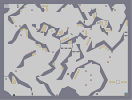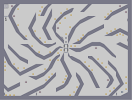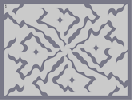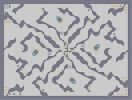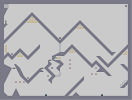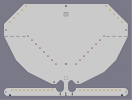Steep Implode Spun Spun In The Hills Landing Strip

Pages: (0)

amazing 5/5

### OMG

Words fail me when i talk about this level. It absolutly superb. I wish i could create something like this.
5/5

### Yea

3.5/5, a bit slow and there aren't many close calls, except at the start.

3/5